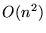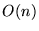Next: Bibliography Up: Crozzle: an NP-Complete Problem Previous: R-BY-C CROZZLE IS      NP-COMPLETE

# SUMMARY

This paper completes the study on the complexity of the Crozzle and R-by-C Crozzle problems (unless a polynomial time algorithm for either is produced). It proves that both problems are NP-Complete. These results build on those of Gower and Wilkerson, who introduced the R-by-C Crozzle problem in order to study the Crozzle problem. They show that the R-by-C Crozzle problem is NP-Hard. The key observation used to demonstrate that R-by-C Crozzle is in NP is the following: since the solution must form a connected unit, the portion of the R-by-C grid that is used is bounded by the size of the input.

To satisfy our sense of curiosity, we ran the Java program discussed in the Appendix on several small 10-by-15 Crozzles, using randomness in place of the non-determinism. The program was run 1,000,000 times on each input.

 Input Input Words 1 book bother keth 2 chemist church sarra 3 active assert movie deduction 4 active assert movie atkinson deduction

 solutions lowest highest Input found score score 1 209 34 50 2 144 40 54 3 5 48 66 4 0 - -

Random placement did not find a solution for any Crozzle with 5 or more words (e.g., Crozzle 4). Solution were found for Crozzles with fewer words. More interesting than the number of solutions is the frequency of their scores. The following table gives the frequency of the scores obtained from Inputs 1 and 2 above.

 Crozzle 1 score 34 36 38 40 42 48 50 frequency 21 36 21 37 60 13 21 Crozzle 2 score 40 32 48 50 54 frequency 19 10 24 31 51

Gower and Wilkerson report that heuristic algorithms designed to solve Crozzles never beat the readers of The Australian Women's Weekly. The above frequencies suggest that the failure of such algorithms may be caused by the high frequency of solutions having the highest score. Thus, the chances of finding a winning solution are comparatively good. In particular, consider Crozzle 2, in which over one of three of the solutions found had the highest score.

APPENDIX

The appendix presents excerpts from a Java program that solves R-by-C Crozzles. The program implements the algorithm from Section 2 except that the non-deterministic choices are replaced by random choices. As seen in Section 3, this is an inefficient approach to solving R-by-C Crozzles. The complete source is presently available at http://www.cs.loyola.edu/`~`binkley/research/Crozzle.

One final note: the complexity of the Java code isbecause the source contains nested loops that examine every square on the grid (e.g., in function score). It is possible to reduce this toby only considering the part of the grid where words are to be placed. For example, initialization would not set all grid squares to blank, but rather it would only initialize those squares where words are to be placed and the squares adjacent to them. Initializing adjacent squares is necessary to check for invalid crozzles (e.g., to check for words that butt end to end.)

```// crozzle.java
// usage: crozzle <file>
// input file format
//   line 1: R, C, word_count
//   rest:   words (one per line)

class InvalidCrozzleException extends Exception {};

class Word
{
public static final int BACK_AND_FORTH = 0;
public static final int UP_AND_DOWN = 1;

protected int row;
protected int column;
protected int orientation;
public String word;

Word(String s)
{
row = -1;
column = -1;
orientation = BACK_AND_FORTH;
word = s;
}

public int length()
{
return(word.length());
}

void assign_random_location(int R, int C)
...
}

class Cell
{
...
}

public class crozzle
{
public static void main(String argv[])
{
RCcrozzle c = new RCcrozzle();
c.place_words();

try
{
System.out.println("score " + c.score()
+ " i = " + i);
}
catch (InvalidCrozzleException e)
{
System.out.println("invalid crozzle");
}
}
}

class RCcrozzle
{
protected int R;
protected int C;
protected Word words[];
protected Cell grid[][];

RCcrozzle()
{
R = 0;
C = 0;
words = null;
}

private int read_int(java.io.StreamTokenizer st)
throws java.io.IOException
{
st.nextToken();
return ((int) st.nval);
}
public void read(java.io.DataInputStream f)
{
int max_word_count = 0;

java.io.StreamTokenizer st
= new java.io.StreamTokenizer(f);
st.parseNumbers();
try
{
}
catch(java.io.IOException e)
{
}

int length = 0;
try
{
words = new Word[max_word_count];

int word_count = 0;
for(int i=0; i<max_word_count; i++)
{
String s = f.readLine();
// using all words now.
// For random inclusion use:
// if (random(2) == 0)
{
words[word_count++] = new Word(s);
length = length + s.length();
}
}
}
catch (java.io.IOException e)
{
}

R = R < length ? R : length;   // bound R and C
C = C < length ? C : length;
}

public void place_words()
{
for(int i=0; i<words.length; i++)
words[i].assign_random_location(R, C);
}

protected int score_for_char(char c)
...

protected boolean two_words_butt()
...

public boolean forms_connected_unit()
...

public int score()
throws InvalidCrozzleException
{
grid = new Cell [R+2][C+2];
for(int i=0; i<R+2; i++)
{
for(int j=0; j<C+2; j++)
grid[i][j] = new Cell('.');
}
...
}

/* returns score for placing letter at a location */
public int place(Cell grid[][], int row,
int column, char c)
throws InvalidCrozzleException
{
if (grid[row][column].empty)
{
grid[row][column].empty = false;
grid[row][column].c = c;
return(0);
}
else if (grid[row][column].c == c)
{
return(score_for_char(c));
}
else  // grid[row][column].c is assigned 2 values
{
throw new InvalidCrozzleException();
}
}
}
```

Next: Bibliography Up: Crozzle: an NP-Complete Problem Previous: R-BY-C CROZZLE IS      NP-COMPLETE